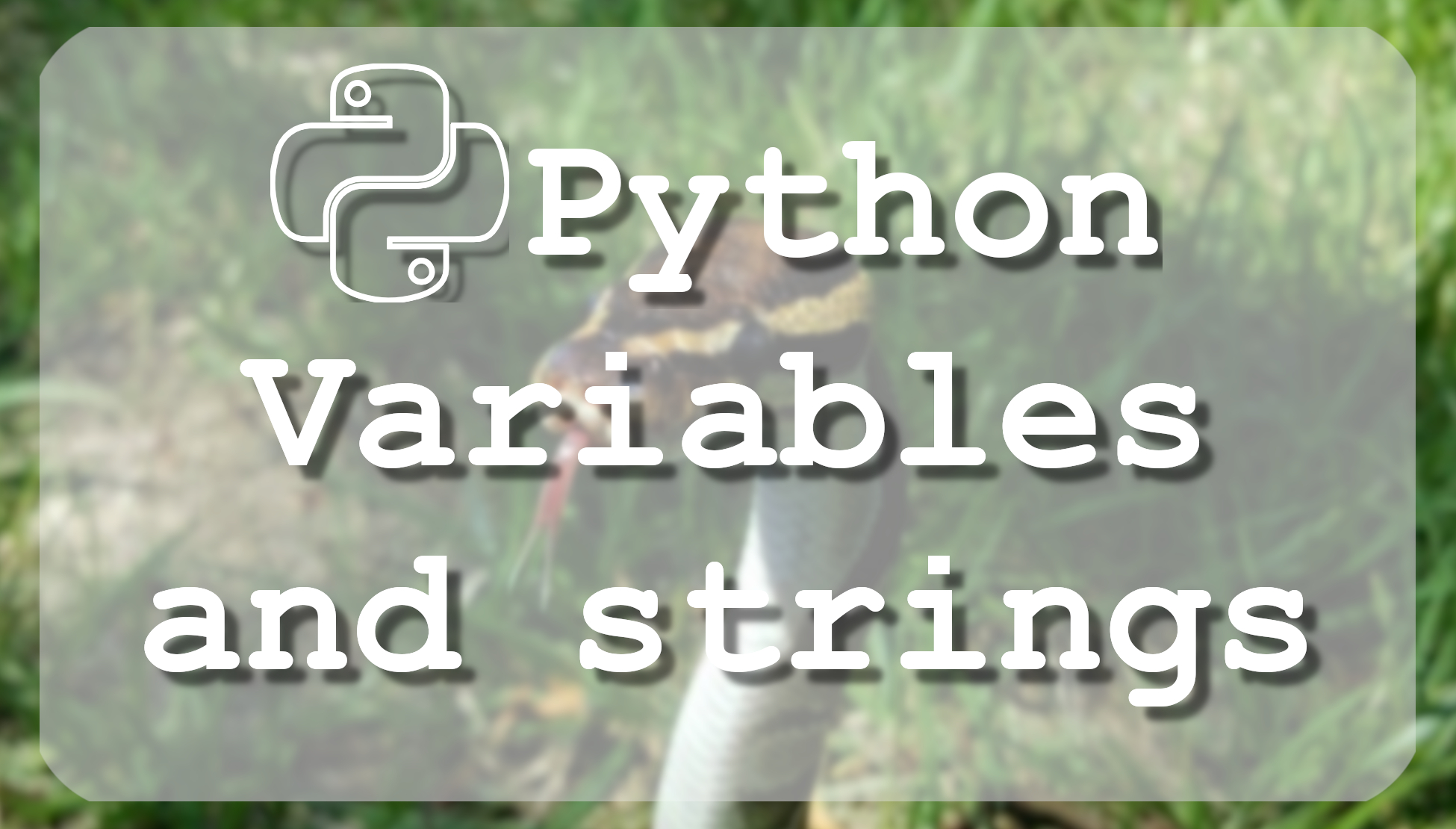# Tutorial - Python 3 basics Python variables and strings

## This tutorial is about python variables and strings. Short introduction with numbers, strings, lists, tuples and dictionaries.

The Most Advanced Data Science Roadmaps You've Ever Seen! Comes with Thousands of Free Learning Resources and ChatGPT Integration! https://aigents.co/learn/roadmaps/intro

In every Python program, you write, you will have variables, which are memory locations to store values. This means that when you create a variable you reserve some space in memory.

Based on the data type of a variable, the interpreter allocates memory and decides what can be stored in the reserved memory. Therefore, by assigning different data types to variables, you can store integers, decimals, or characters.

Stored data in memory can be of many types. For example, a person's age can be stored as a numeric value and his or her email or address can be stored as alphanumeric characters.
Python has five standard data types that are used to define the operations possible on them and the storage method for each of them.
Five standard data types:

• Numbers

• Strings

• List

• Tuple

• Dictionary

In Python, variables don't need explicit declaration (declared number will be float or long integer). The declaration happens automatically when you assign a value to a variable. The operand to the left of the = operator is the name of the variable and the operand to the right of the = operator is the value stored in the variable, for example:

```hundret  = 100          # An integer assignment
thousand = 1000.0       # A floating point
name     = "Tomm"       # A string
surname  = "Gates"      # A string```

Python allows using multiple assignments, it means you can assign a single value to several variables simultaneously. Example:

`a = b = c = number = 7`

Here, an integer object is created with the value 7, and all three variables are assigned to the same memory location. By the way, you can also assign multiple objects to multiple variables. Example:

`a, b, c, number = 7,9,"Tomm", "2018"`

## Python Numbers:

Number data types store numeric values. Number objects are created when you assign a value to them, as we already mentioned. But we didn't mention that Python supports four different numerical types:

• Int (signed integers), its default number;

• Long (long integers), to the end of number add letter L, for example: 131752361L or 0xDEFABCECBDAECBFBAEl;

• Float (floating point real values), the number becomes float when you give him numbers with comma, for example, -21.9 or 32.3+e18;

• Complex (complex numbers);

More difficult is with a complex number which consists of an ordered pair of real floating-point numbers denoted by x+yj, where x and y are the real numbers and j is the imaginary unit. But we almost never use Complex numbers.

## Python Strings:

Strings in Python are identified as a contiguous set of characters represented in the quotation marks. In the previous tutorial, we mentioned that Python allows pairs of single or double-quotes. The subsets of strings can be taken using the slice operator ([ ] and [:]) with indexes starting at 0 at the beginning of the string and working their way from -1 at the end. Example:

``````string = 'Welcome to my World!'

print (string)           # Prints complete string
print (string)        # Prints first character of the string
print (string[2:10])     # Prints characters starting from 3rd to 11th
print (string[8:])       # Prints string starting from 9rd character
print (string * 2)       # Prints string two times
print (string + " TEST") # Prints concatenated string``````

The plus (+) sign is the string concatenation operator and the asterisk (*) is the repetition operator.

In a print Shell this will produce the following result:

```Welcome to my World!
W
lcome to
to my World!
Welcome to my World!Welcome to my World!
Welcome to my World! TEST```

Now we covered the basics of variables and strings. There is more to learn about variables, for example, we can use them locally or globally, but this will be covered in a future tutorial.